# The ggplot2 plotting system: ggplot()

An overview of the ggplot2 plotting system in R with ggplot().

Stephanie Hicks https://stephaniehicks.com/ (Department of Biostatistics, Johns Hopkins)https://www.jhsph.edu
09-14-2021

# Pre-lecture materials

Before class, you can prepare by reading the following materials:

### Acknowledgements

Material for this lecture was borrowed and adopted from

# Learning objectives

At the end of this lesson you will:

• Be able to build up layers of graphics using `ggplot()`
• Be able to modify properties of a `ggplot()` including layers and labels

# The ggplot2 Plotting System

In this lesson, we will get into a little more of the nitty gritty of how `ggplot2` builds plots and how you can customize various aspects of any plot.

In the previous lesson, we used the `qplot()` function to quickly put points on a page. The `qplot()` function’s syntax is very similar to that of the `plot()` function in base graphics so for those switching over, it makes for an easy transition. But it is worth knowing the underlying details of how `ggplot2` works so that you can really exploit its power.

### Basic components of a ggplot2 plot

A `ggplot2` plot consists of a number of key components. Here are a few of the more commonly used ones.

• A data frame: stores all of the data that will be displayed on the plot

• aesthetic mappings: describe how data are mapped to color, size, shape, location

• geoms: geometric objects like points, lines, shapes.

• facets: describes how conditional/panel plots should be constructed

• stats: statistical transformations like binning, quantiles, smoothing.

• scales: what scale an aesthetic map uses (example: left-handed = red, right-handed = blue).

• coordinate system: describes the system in which the locations of the geoms will be drawn

It is essential that you properly organize your data into a data frame before you start with `ggplot2`. In particular, it is important that you provide all of the appropriate metadata so that your data frame is self-describing and your plots will be self-documenting.

When building plots in ggplot2 (rather than using `qplot()`), the “artist’s palette” model may be the closest analogy. Essentially, you start with some raw data, and then you gradually add bits and pieces to it to create a plot. Plots are built up in layers, with the typically ordering being

1. Plot the data

2. Overlay a summary

For quick exploratory plots you may not get past step 1.

### Example: BMI, PM2.5, Asthma

To demonstrate the various pieces of `ggplot2` we will use a running example from the Mouse Allergen and Asthma Cohort Study (MAACS), which was used as a case study in the previous lesson. Here, the question we are interested in is

“Are overweight individuals, as measured by body mass index (BMI), more susceptible than normal weight individuals to the harmful effects of PM2.5 on asthma symptoms?”

There is a suggestion that overweight individuals may be more susceptible to the negative effects of inhaling PM2.5. This would suggest that increases in PM2.5 exposure in the home of an overweight child would be more deleterious to his/her asthma symptoms than they would be in the home of a normal weight child. We want to see if we can see that difference in the data from MAACS.

Because the individual-level data for this study are protected by various U.S. privacy laws, we cannot make those data available. For the purposes of this lesson, we have simulated data that share many of the same features of the original data, but do not contain any of the actual measurements or values contained in the original dataset.

We can look at the data quickly by reading it in as a tibble with `read_csv()` in the `tidyverse` package.

``````library(tidyverse)
library(here)
col_types = "nnci")
maacs
``````
``````# A tibble: 517 × 4
logpm25 logno2_new bmicat        NocturnalSympt
<dbl>      <dbl> <chr>                  <int>
1   1.25       1.18  normal weight              1
2   1.12       1.55  overweight                 0
3   1.93       1.43  normal weight              0
4   1.37       1.77  overweight                 2
5   0.775      0.765 normal weight              0
6   1.49       1.11  normal weight              0
7   2.16       1.43  normal weight              0
8   1.65       1.40  normal weight              0
9   1.55       1.81  normal weight              0
10   2.04       1.35  overweight                 3
# … with 507 more rows``````

The outcome we will look at here, `NocturnalSymp`, is the number of days in the past 2 weeks where the child experienced asthma symptoms (e.g. coughing, wheezing) while sleeping.

The other key variables are:

• `logpm25`: average level of PM2.5 over the course of 7 days (micrograms per cubic meter) on the log scale

• `logno2_new`: exhaled nitric oxide on the log scale

• `bmicat`: categorical variable with BMI status

### Building up in layers

First, we can create a `ggplot` object that stores the dataset and the basic aesthetics for mapping the x- and y-coordinates for the plot. Here, we will eventually be plotting the log of PM2.5 and `NocturnalSymp` variable.

``````g <- ggplot(maacs, aes(x = logpm25, y = NocturnalSympt))
summary(g)
``````
``````data: logpm25, logno2_new, bmicat, NocturnalSympt [517x4]
mapping:  x = ~logpm25, y = ~NocturnalSympt
faceting: <ggproto object: Class FacetNull, Facet, gg>
compute_layout: function
draw_back: function
draw_front: function
draw_labels: function
draw_panels: function
finish_data: function
init_scales: function
map_data: function
params: list
setup_data: function
setup_params: function
shrink: TRUE
train_scales: function
vars: function
super:  <ggproto object: Class FacetNull, Facet, gg>``````
``````class(g)
``````
`` "gg"     "ggplot"``

You can see above that the object `g` contains the dataset `maacs` and the mappings.

Now, normally if you were to `print()` a `ggplot` object a plot would appear on the plot device, however, our object `g` actually does not contain enough information to make a plot yet.

``````g <- maacs %>%
ggplot(aes(logpm25, NocturnalSympt))
print(g)
``````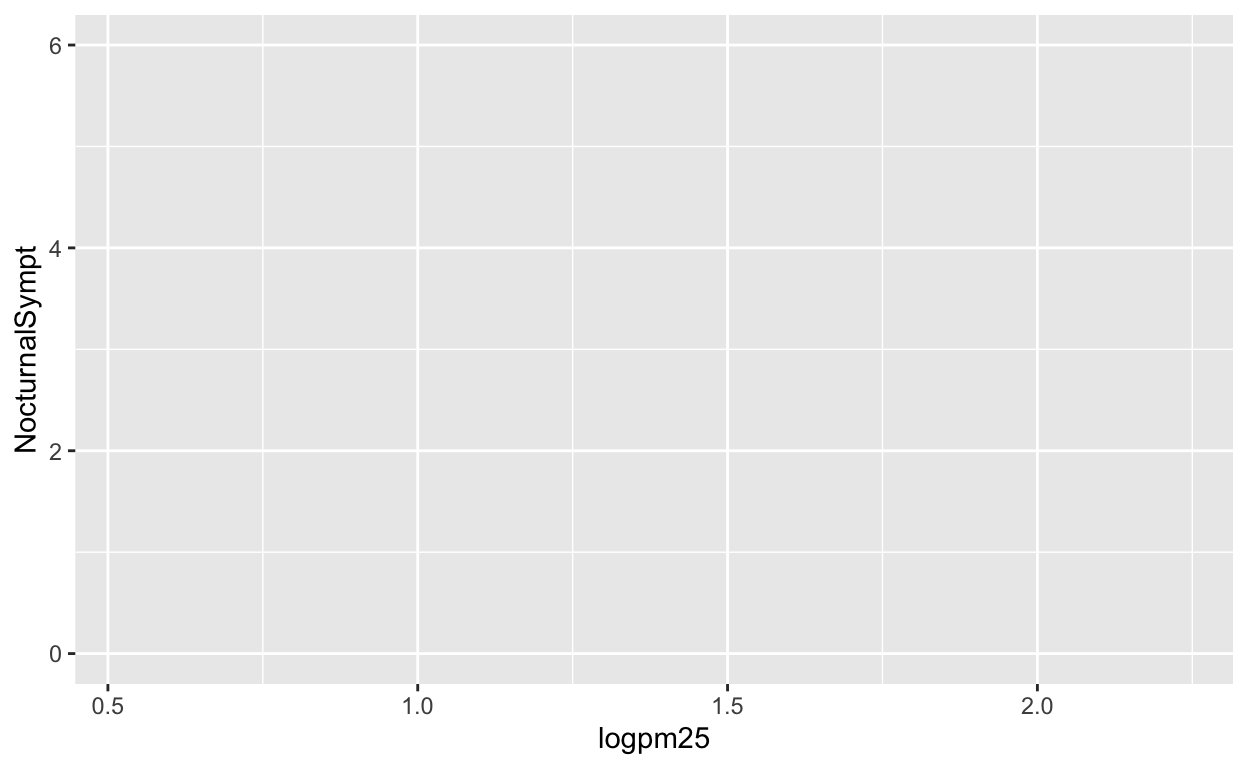Figure 1: Nothing to see here!

### First plot with point layer

To make a scatterplot we need add at least one geom, such as points. Here, we add the `geom_point()` function to create a traditional scatterplot.

``````g <- maacs %>%
ggplot(aes(logpm25, NocturnalSympt))
g + geom_point()
``````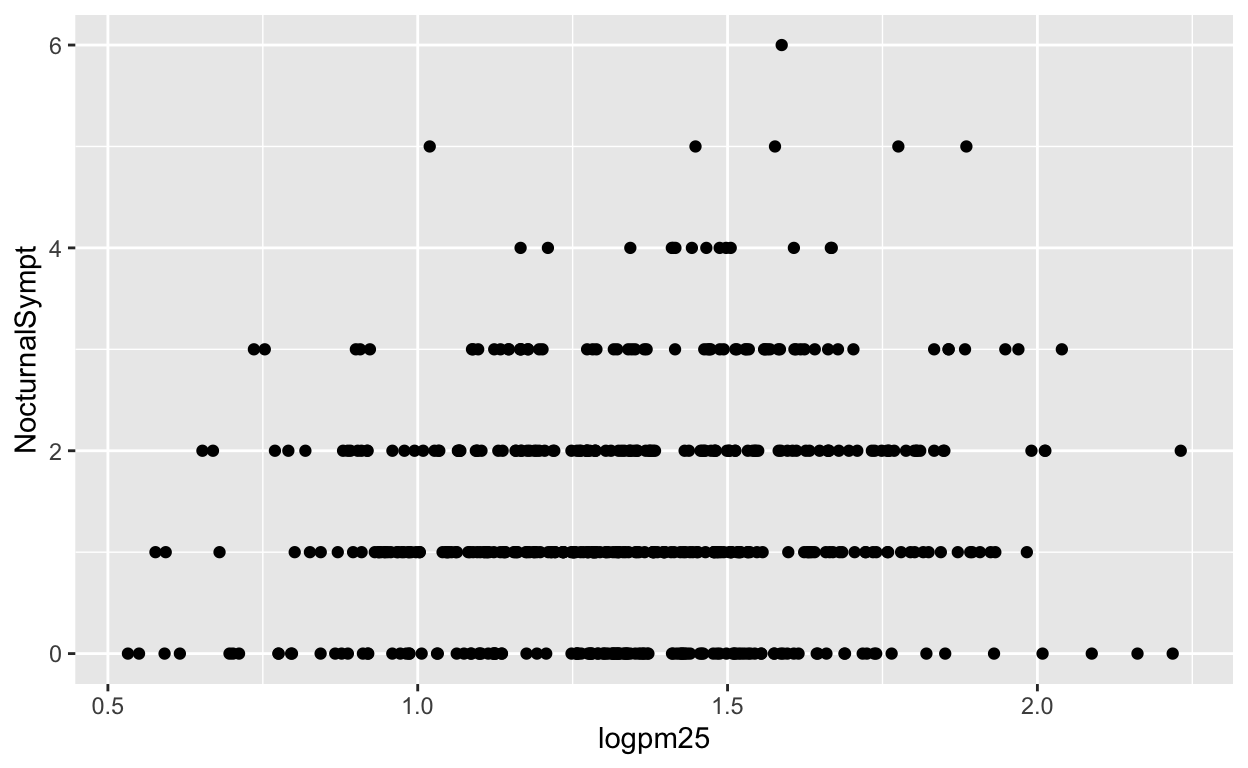How does ggplot know what points to plot? In this case, it can grab them from the data frame `maacs` that served as the input into the `ggplot()` function.

Because the data appear rather noisy, it might be better if we added a smoother on top of the points to see if there is a trend in the data with PM2.5.

``````g + geom_point() + geom_smooth()
``````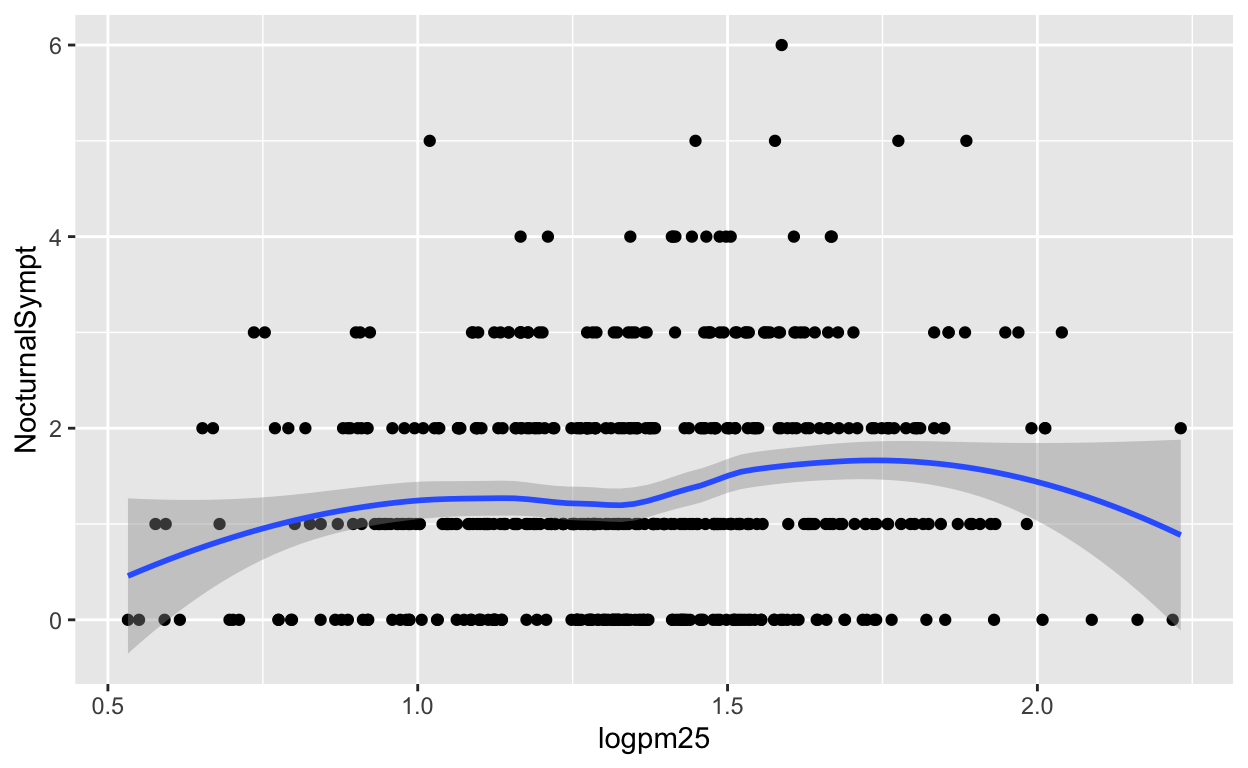Figure 2: Scatterplot with smoother

The default smoother is a loess smoother, which is flexible and nonparametric but might be too flexible for our purposes. Perhaps we’d prefer a simple linear regression line to highlight any first order trends. We can do this by specifying `method = "lm"` to `geom_smooth()`.

``````g + geom_point() + geom_smooth(method = "lm")
``````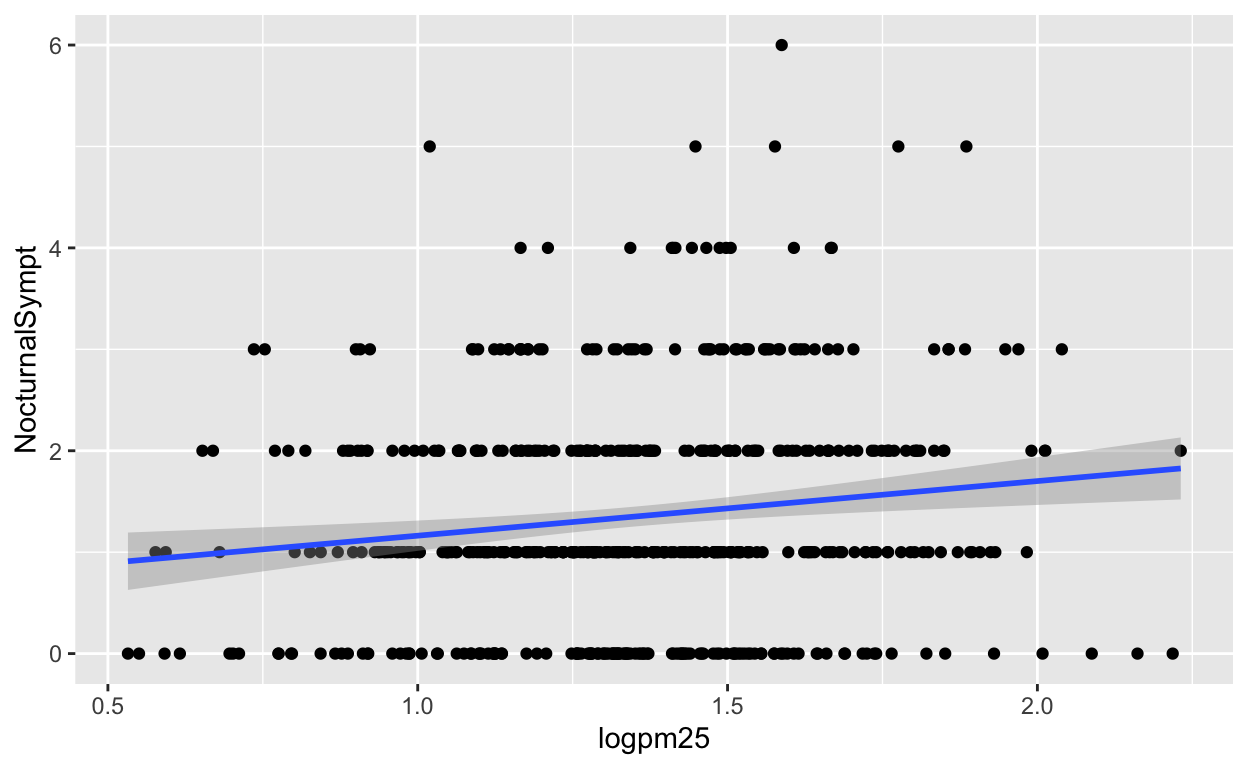Figure 3: Scatterplot with linear regression line

Here, we can see there appears to be a slight increasing trend, suggesting that higher levels of PM2.5 are associated with increased days with nocturnal symptoms.

Example: Let’s use the `ggplot()` function with our `palmerpenguins` dataset example and make a scatter plot with `flipper_length_mm` on the x-axis, `bill_length_mm` on the y-axis, colored by `species`, and a smoother by adding a linear regression.
``````# try it yourself

library(palmerpenguins)
penguins
``````
``````# A tibble: 344 × 8
species island    bill_length_mm bill_depth_mm flipper_length_mm
<fct>   <fct>              <dbl>         <dbl>             <int>
1 Adelie  Torgersen           39.1          18.7               181
2 Adelie  Torgersen           39.5          17.4               186
3 Adelie  Torgersen           40.3          18                 195
4 Adelie  Torgersen           NA            NA                  NA
5 Adelie  Torgersen           36.7          19.3               193
6 Adelie  Torgersen           39.3          20.6               190
7 Adelie  Torgersen           38.9          17.8               181
8 Adelie  Torgersen           39.2          19.6               195
9 Adelie  Torgersen           34.1          18.1               193
10 Adelie  Torgersen           42            20.2               190
# … with 334 more rows, and 3 more variables: body_mass_g <int>,
#   sex <fct>, year <int>``````

Because our primary question involves comparing overweight individuals to normal weight individuals, we can stratify the scatter plot of PM2.5 and nocturnal symptoms by the BMI category (`bmicat`) variable, which indicates whether an individual is overweight or now. To visualize this we can add a `facet_grid()`, which takes a formula argument. Here we want one row and two columns, one column for each weight category. So we specify `bmicat` on the right hand side of the forumla passed to `facet_grid()`.

``````g + geom_point() +
geom_smooth(method = "lm") +
facet_grid(. ~ bmicat)
``````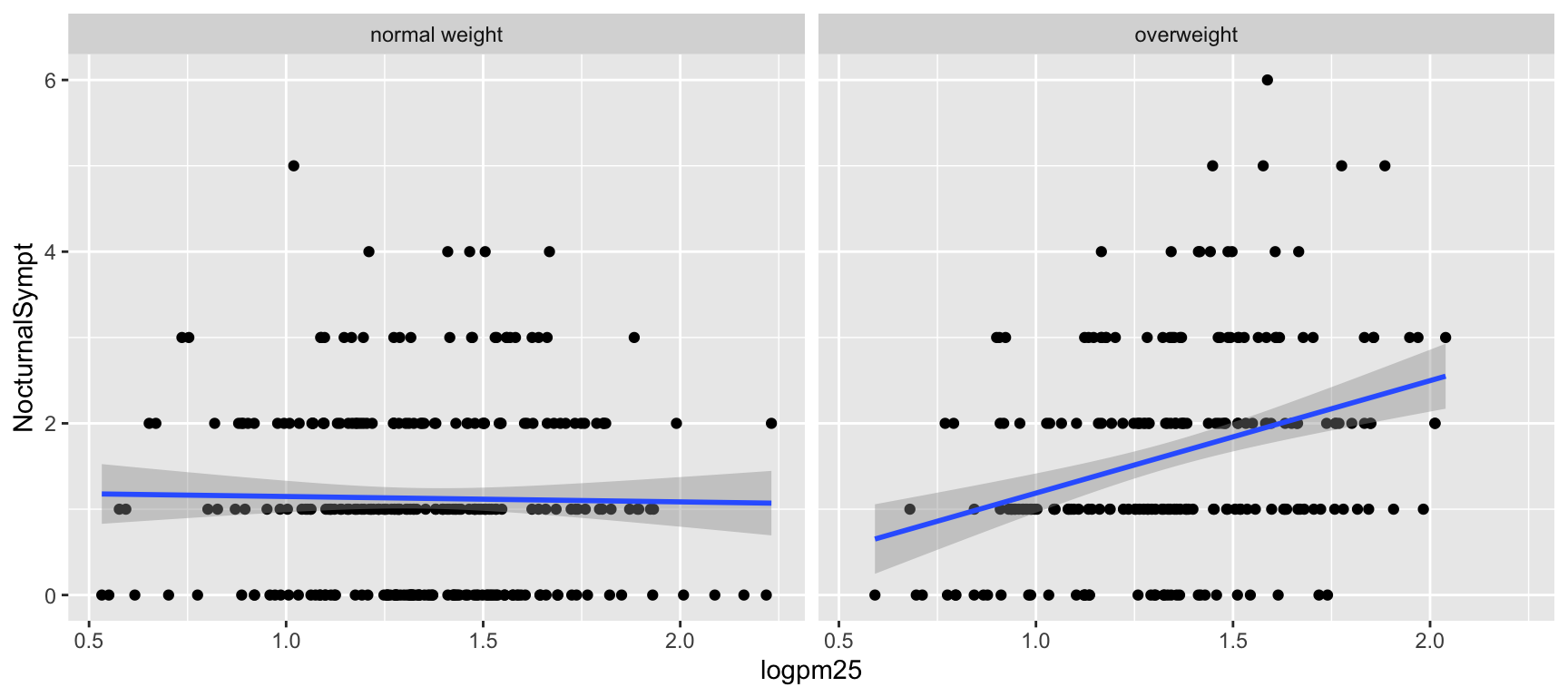Figure 4: Scatterplot of PM2.5 and nocturnal symptoms by BMI category

Now it seems clear that the relationship between PM2.5 and nocturnal symptoms is relatively flat among normal weight individuals, while the relationship is increasing among overweight individuals. This plot suggests that overweight individuals may be more susceptible to the effects of PM2.5.

There are a variety of annotations you can add to a plot, including different kinds of labels. You can use `xlab()` for x-axis labels, `ylab()` for y-axis labels, and `ggtitle()` for specifying plot titles. The `labs()` function is generic and can be used to modify multiple types of labels at once.

For things that only make sense globally, use `theme()`, i.e. `theme(legend.position = "none")`. Two standard appearance themes are included

• `theme_gray()`: The default theme (gray background)

• `theme_bw()`: More stark/plain

### Modifying geom properties

You can modify properties of geoms by specifying options to their respective `geom_*` functions. For example, here we modify the points in the scatterplot to make the color “steelblue”, the size larger , and the alpha transparency greater.

``````g + geom_point(color = "steelblue", size = 4, alpha = 1/2)
``````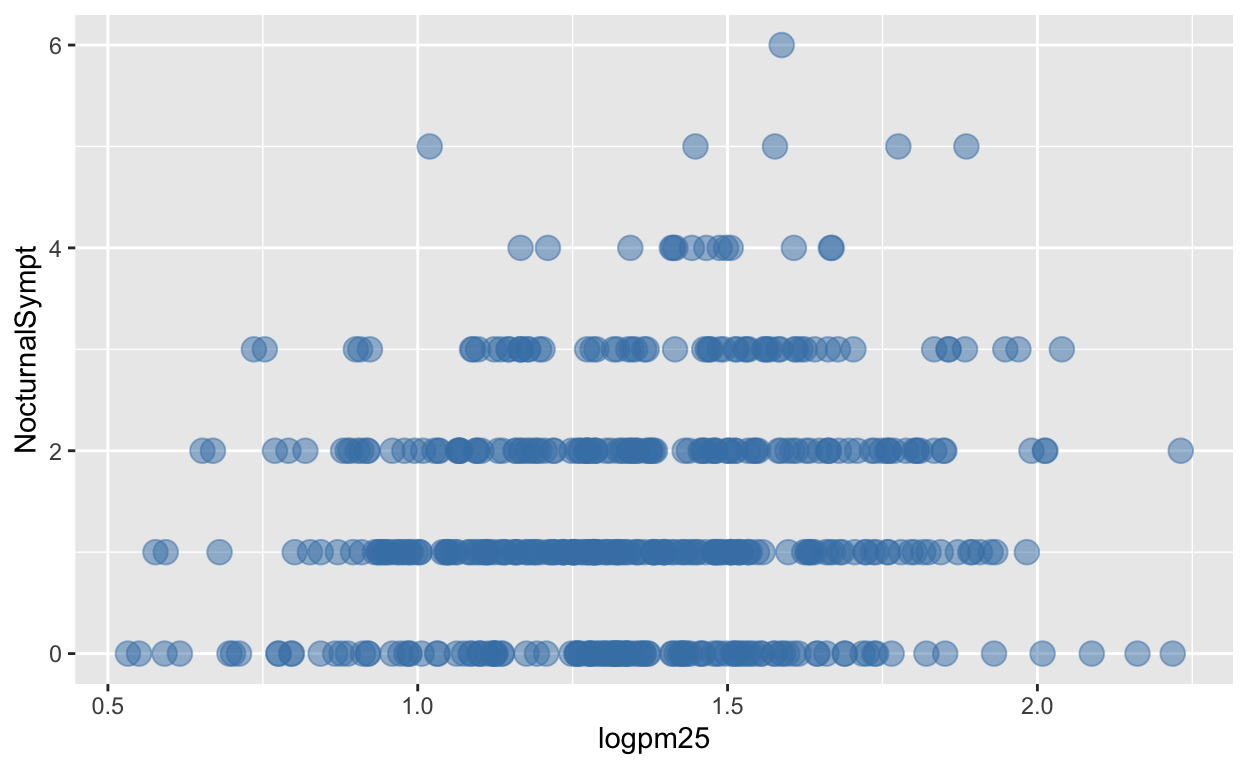Figure 5: Modifying point color with a constant

In addition to setting specific geom attributes to constants, we can map aesthetics to variables. So, here, we map the color aesthetic `color` to the variable `bmicat`, so the points will be colored according to the levels of `bmicat`. We use the `aes()` function to indicate this difference from the plot above.

``````g + geom_point(aes(color = bmicat), size = 4, alpha = 1/2)
``````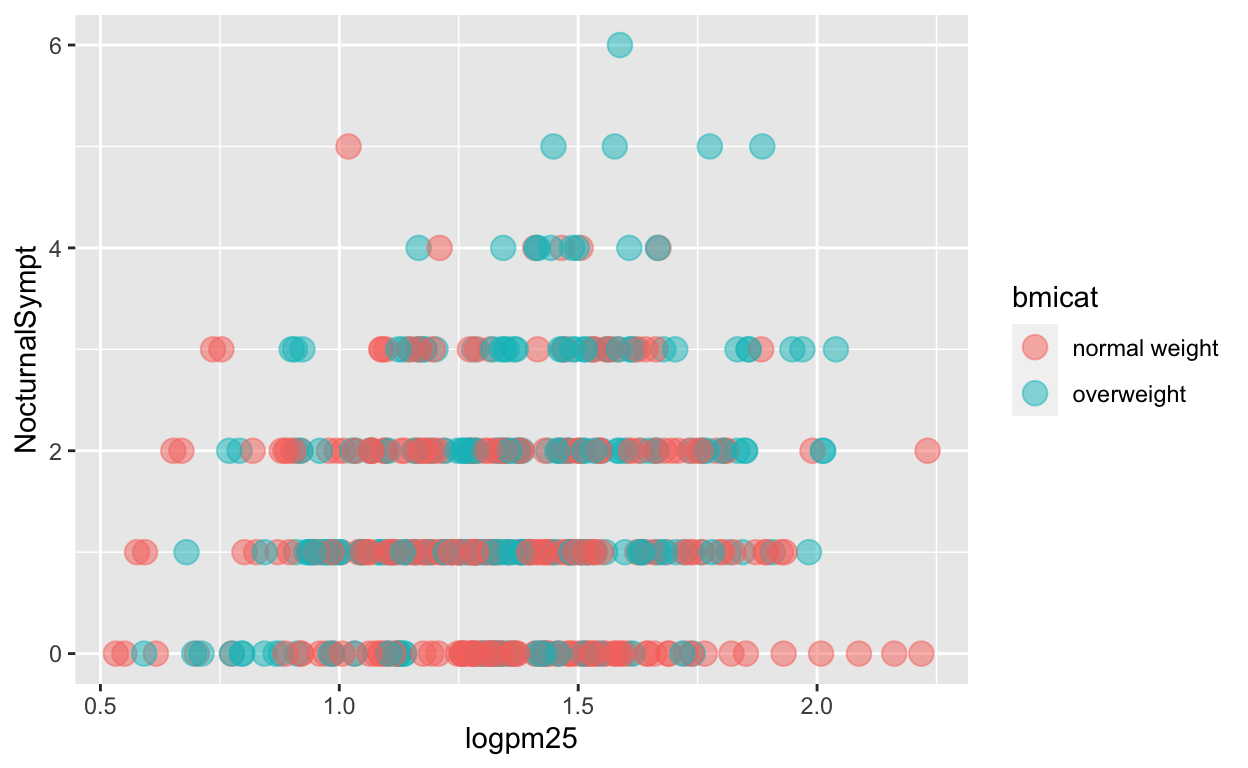Figure 6: Mapping color to a variable

### Modifying labels

Here is an example of modifying the title and the x and y labels to make the plot a bit more informative.

``````g + geom_point(aes(color = bmicat)) +
labs(title = "MAACS Cohort") +
labs(x = expression("log " * PM[2.5]), y = "Nocturnal Symptoms")
``````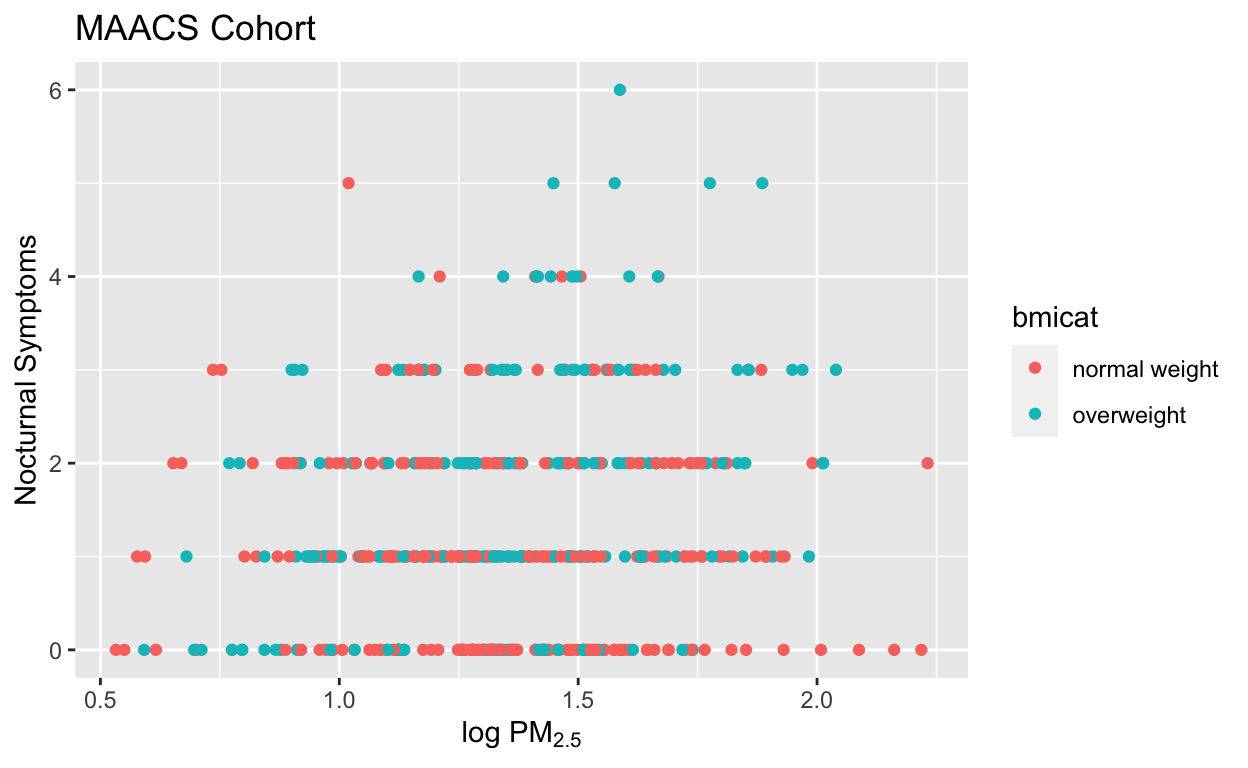Figure 7: Modifying plot labels

### Customizing the smooth

We can also customize aspects of the smoother that we overlay on the points with `geom_smooth()`. Here we change the line type and increase the size from the default. We also remove the shaded standard error from the line.

``````g + geom_point(aes(color = bmicat),
size = 2, alpha = 1/2) +
geom_smooth(size = 4, linetype = 3,
method = "lm", se = FALSE)
``````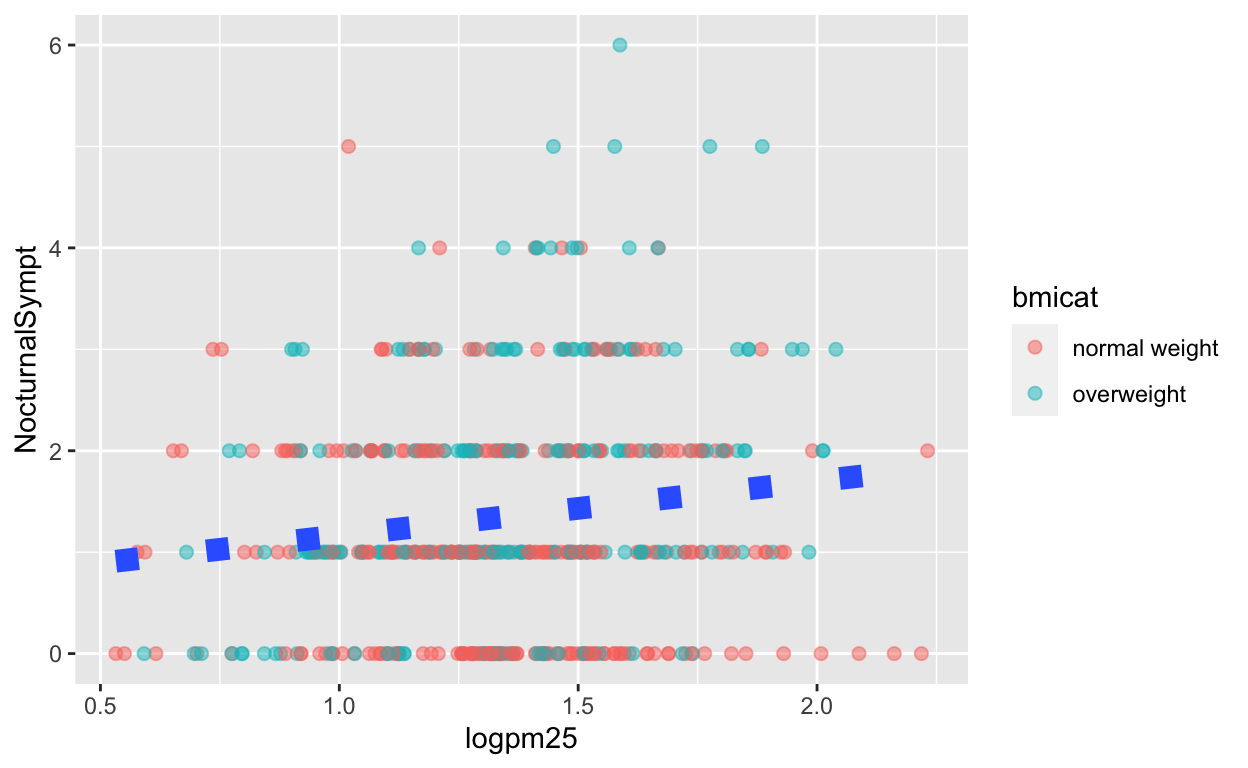Figure 8: Customizing a smoother

### Changing the theme

The default theme for `ggplot2` uses the gray background with white grid lines. If you don’t find this suitable, you can use the black and white theme by using the `theme_bw()` function. The `theme_bw()` function also allows you to set the typeface for the plot, in case you don’t want the default Helvetica. Here we change the typeface to Times.

``````g + geom_point(aes(color = bmicat)) +
theme_bw(base_family = "Times")
``````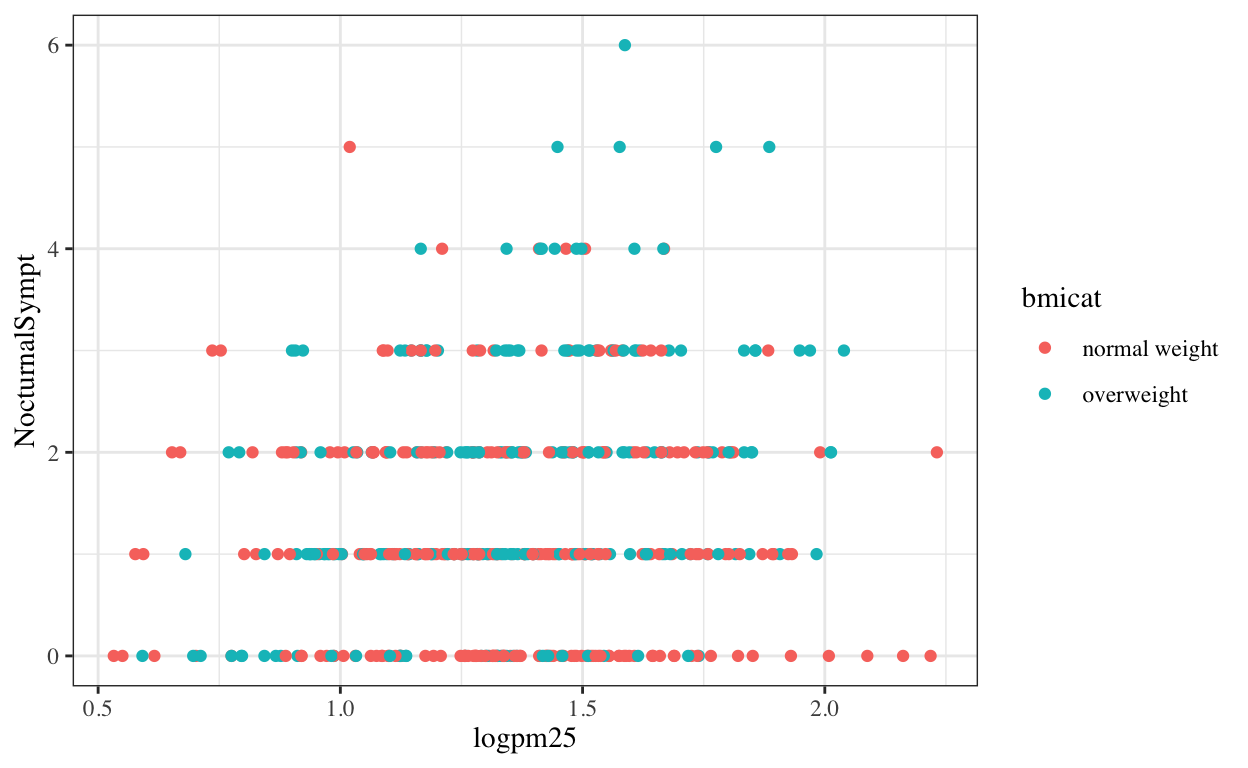Figure 9: Modifying the theme for a plot

Example: Let’s take our `palmerpenguins` scatterplot from above and change out the theme to use `theme_dark()`.
``````# try it yourself

library(palmerpenguins)
penguins
``````
``````# A tibble: 344 × 8
species island    bill_length_mm bill_depth_mm flipper_length_mm
<fct>   <fct>              <dbl>         <dbl>             <int>
1 Adelie  Torgersen           39.1          18.7               181
2 Adelie  Torgersen           39.5          17.4               186
3 Adelie  Torgersen           40.3          18                 195
4 Adelie  Torgersen           NA            NA                  NA
5 Adelie  Torgersen           36.7          19.3               193
6 Adelie  Torgersen           39.3          20.6               190
7 Adelie  Torgersen           38.9          17.8               181
8 Adelie  Torgersen           39.2          19.6               195
9 Adelie  Torgersen           34.1          18.1               193
10 Adelie  Torgersen           42            20.2               190
# … with 334 more rows, and 3 more variables: body_mass_g <int>,
#   sex <fct>, year <int>``````

### More complex example

Now you get the sense that plots in the `ggplot2` system are constructed by successively adding components to the plot, starting with the base dataset and maybe a scatterplot. In this section bleow, you can see a slightly more complicated example with an additional variable.

Click here for a slightly more complicated example with `ggplot()`.

Now, we will ask the question

How does the relationship between PM2.5 and nocturnal symptoms vary by BMI category and nitrogen dioxide (NO2)?

Unlike our previous BMI variable, NO2 is continuous, and so we need to make NO2 categorical so we can condition on it in the plotting. We can use the `cut()` function for this purpose. We will divide the NO2 variable into tertiles.

First we need to calculate the tertiles with the `quantile()` function.

``````cutpoints <- quantile(maacs\$logno2_new, seq(0, 1, length = 4), na.rm = TRUE)
``````

Then we need to divide the original `logno2_new` variable into the ranges defined by the cut points computed above.

``````maacs\$no2tert <- cut(maacs\$logno2_new, cutpoints)
``````

The `not2tert` variable is now a categorical factor variable containing 3 levels, indicating the ranges of NO2 (on the log scale).

``````## See the levels of the newly created factor variable
levels(maacs\$no2tert)
``````
`` "(0.342,1.23]" "(1.23,1.47]"  "(1.47,2.17]" ``

The final plot shows the relationship between PM2.5 and nocturnal symptoms by BMI category and NO2 tertile.

``````## Setup ggplot with data frame
g <- maacs %>%
ggplot(aes(logpm25, NocturnalSympt))

g + geom_point(alpha = 1/3) +
facet_grid(bmicat ~ no2tert) +
geom_smooth(method="lm", se=FALSE, col="steelblue") +
theme_bw(base_family = "Avenir", base_size = 10) +
labs(x = expression("log " * PM[2.5])) +
labs(y = "Nocturnal Symptoms") +
labs(title = "MAACS Cohort")
``````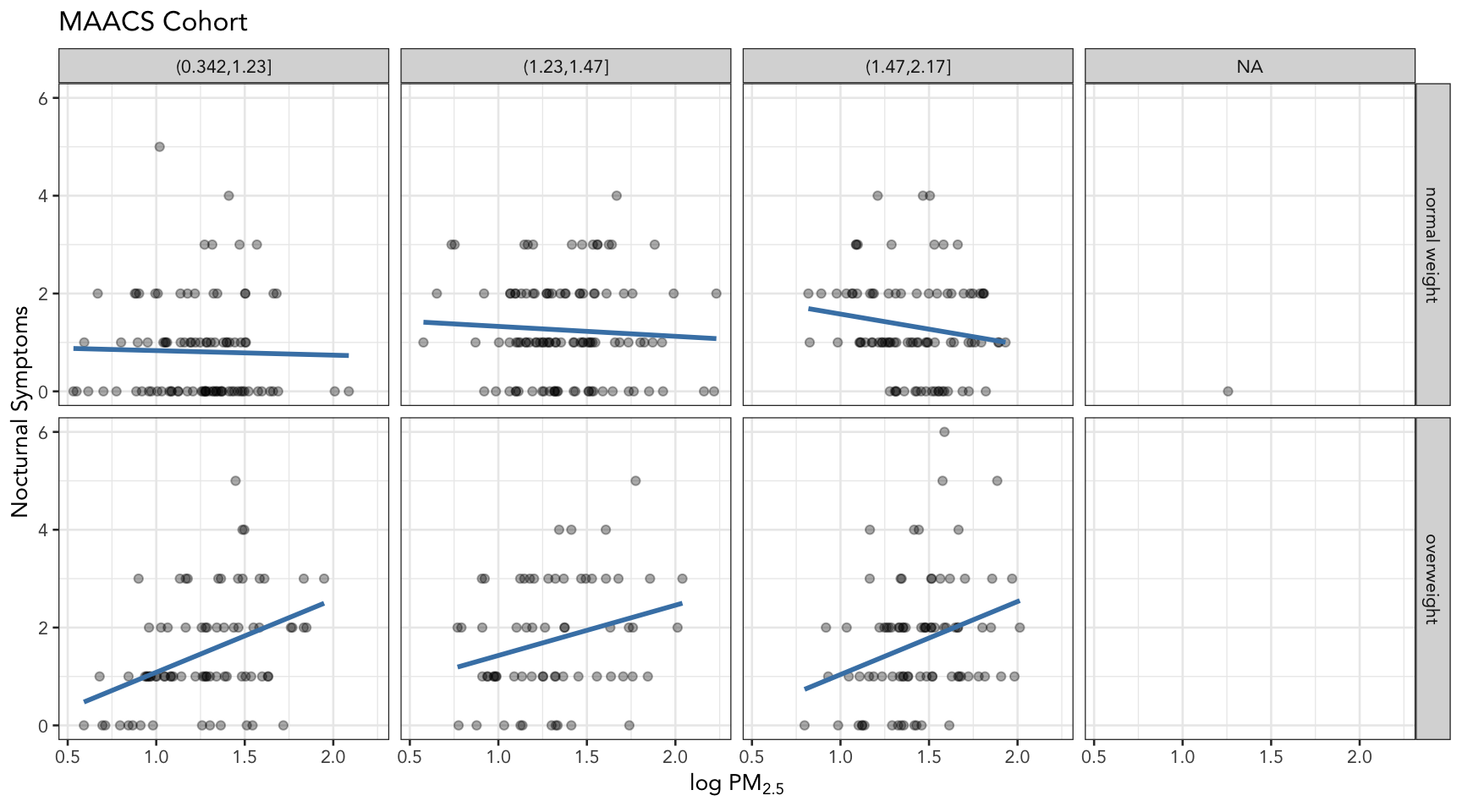Figure 10: PM2.5 and nocturnal symptoms by BMI category and NO2 tertile

## A quick aside about axis limits

One quick quirk about `ggplot2` that caught me up when I first started using the package can be displayed in the following example. I make a lot of time series plots and I often want to restrict the range of the y-axis while still plotting all the data. In the base graphics system you can do that as follows.

``````testdat <- data.frame(x = 1:100, y = rnorm(100))
testdat[50,2] <- 100  ## Outlier!
plot(testdat\$x, testdat\$y, type = "l", ylim = c(-3,3))
``````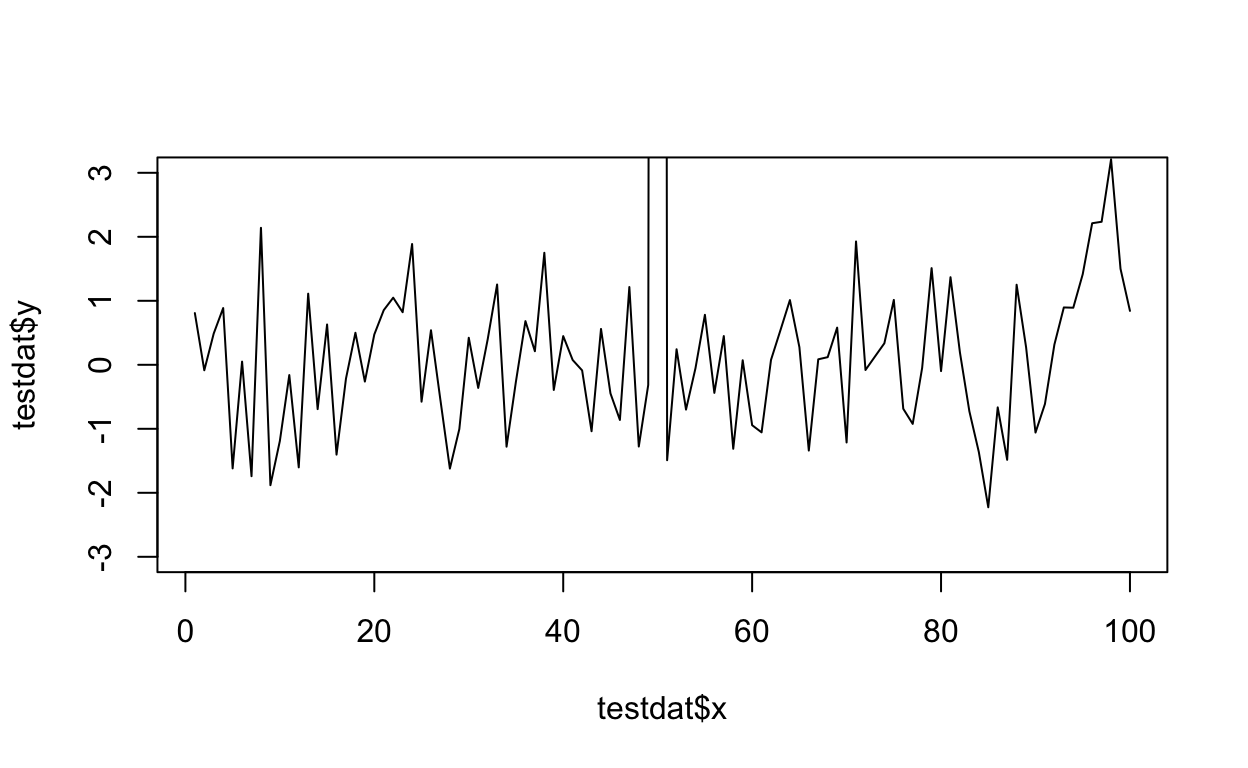Figure 11: Time series plot with base graphics

Here I’ve restricted the y-axis range to be between -3 and 3, even though there is a clear outlier in the data.

With `ggplot2` the default settings will give you this.

``````g <- ggplot(testdat, aes(x = x, y = y))
g + geom_line()
``````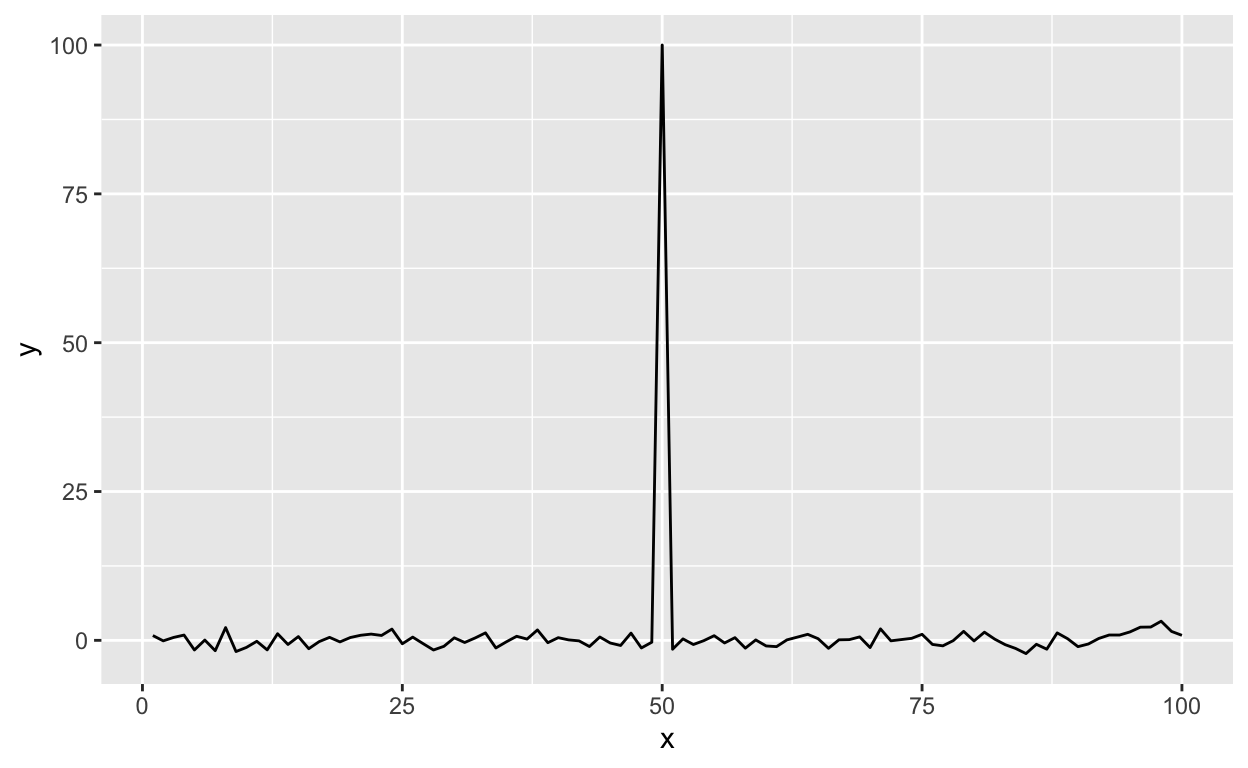Figure 12: Time series plot with default settings

Modifying the `ylim()` attribute would seem to give you the same thing as the base plot, but it doesn’t.

``````g + geom_line() + ylim(-3, 3)
``````Figure 13: Time series plot with modified ylim

Effectively, what this does is subset the data so that only observations between -3 and 3 are included, then plot the data.

To plot the data without subsetting it first and still get the restricted range, you have to do the following.

``````g + geom_line() + coord_cartesian(ylim = c(-3, 3))
``````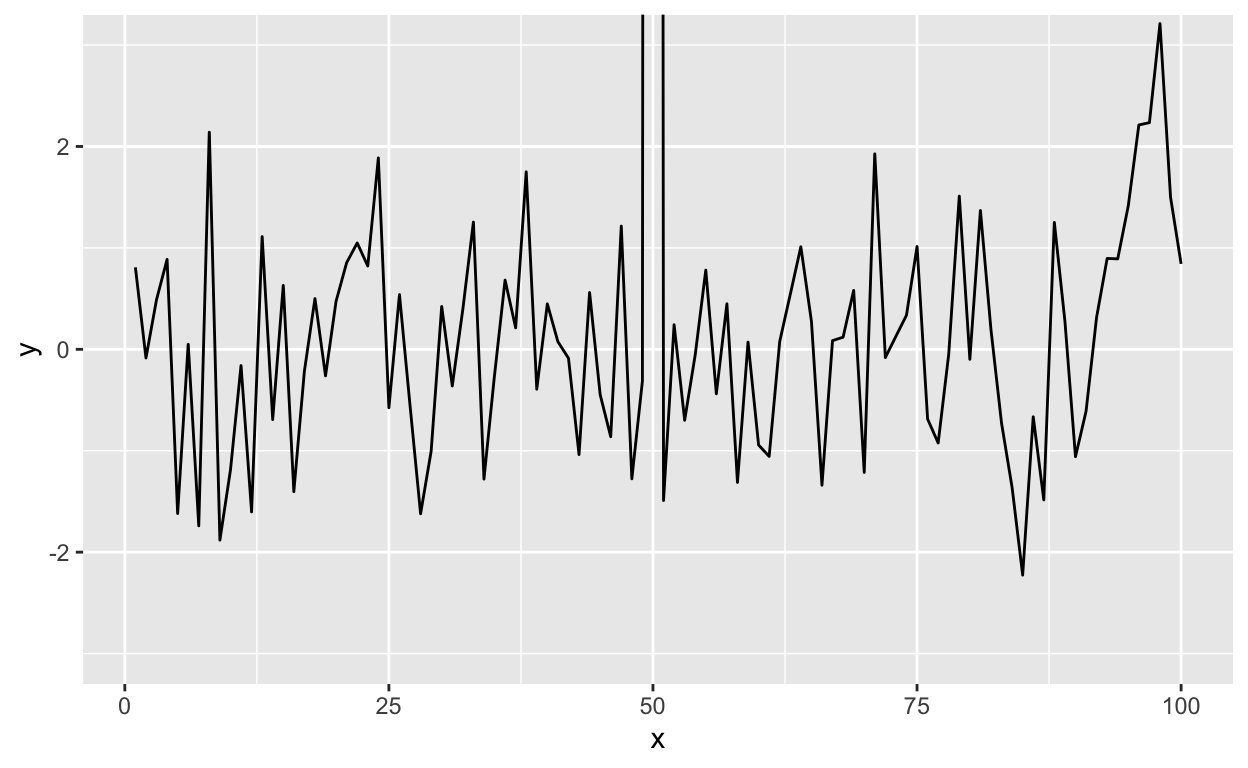Figure 14: Time series plot with restricted y-axis range

And now you know!

### Resources

• The ggplot2 book by Hadley Wickham
• The R Graphics Cookbook by Winston Chang (examples in base plots and in ggplot2)
• tidyverse web site

# Post-lecture materials

### Final Questions

Questions:

1. What happens if you facet on a continuous variable?

2. Read `?facet_wrap`. What does `nrow` do? What does `ncol` do? What other options control the layout of the individual panels? Why doesn’t `facet_grid()` have `nrow` and `ncol` arguments?

3. What geom would you use to draw a line chart? A boxplot? A histogram? An area chart?

4. What does `geom_col()` do? How is it different to `geom_bar()`?

### Reuse

Text and figures are licensed under Creative Commons Attribution CC BY-NC-SA 4.0. The figures that have been reused from other sources don't fall under this license and can be recognized by a note in their caption: "Figure from ...".

### Citation

`Hicks (2021, Sept. 14). Statistical Computing: The ggplot2 plotting system: ggplot(). Retrieved from https://stephaniehicks.com/jhustatcomputing2021/posts/2021-09-14-ggplot2-plotting-system-part-2/`
```@misc{hicks2021the,